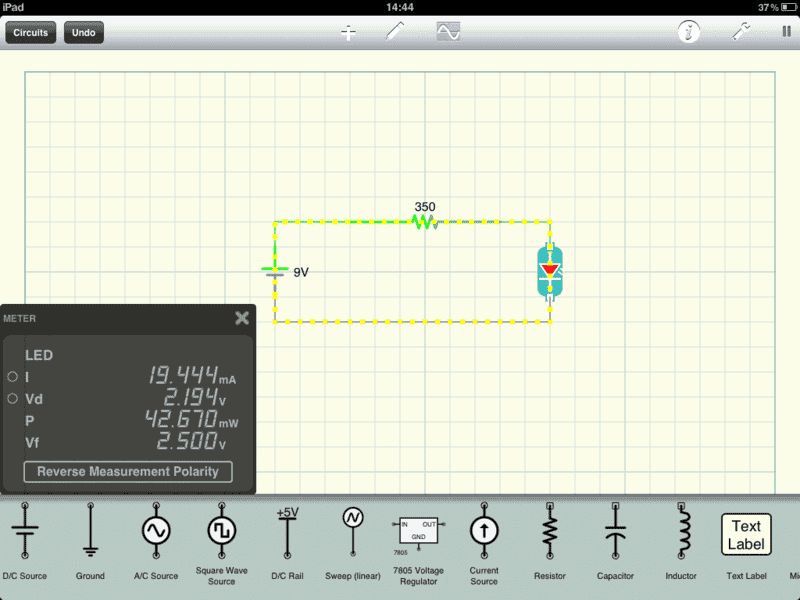# Forward Voltage / Dropped Voltage / LEDs

• Irishwake

#### Irishwake

Hiya,

A co-worker and I were a little bored at work the last week and stumbled our way into playing around with basic circuits. Long story short we have made several simple series and parallel circuit diagrams and we practice working out the math and voltage drops across resistors and what not.

A question we cannot seem to be definitively answered is this; What is the relationship of Voltage Dropped across the LED and the LEDs rated Forward Voltage? Let's say we have a simple circuit:

9V DC Power Source > 350Ω Resistor > Single Red LED with Vf of 2.5V

First we calculated (based on the data sheet of the LED stating 20mA for I) that we would need a 325Ω resistor. (I used a 350Ω above to get the current under the LED rating, 19.444mA specifically).

This gives us a voltage drop of 6.806V at the resistor and 2.194V at the LED.

What is the relationship of Voltage Dropped across the LED and the LEDs rated Forward Voltage?

Does the Vd across the LED being LESS than the Vf rating of the LED have anything to do with, well anything?

What would happen if Vd was more than Vf? Would the LED just burn itself out?

Does Vd need to be less than Vf?

Thanks in advance for what seem to be some simple questions that we're having difficulty getting straight answers to.

The data sheet is telling you that a current through the LED of 20 mA will develop a voltage of 2.5 volts across the LED. You are wondering what happens when you set up a circuit as described above in that you have reduced the current correct? Diodes are not linear devices so you really don't know. All you know is that when extra resistance is introduced into the circuit (350 instead of 325) the current will drop and so will the voltage across the LED. But they will not track linearly. Also, realize that the data sheet specs are not cast in stone. They can change with temperature and from one LED to the next. After all, they really don't need to be all that precise. They are indicators and the specs give the designer a place to start at in resistor selection and usually the arrival at that resistor value is not tweaked.

The data sheet is telling you that a current through the LED of 20 mA will develop a voltage of 2.5 volts across the LED.

That is exactly what I was looking for.

I'm using iCircuit on my iPad to draw out my circuits to check my math and it seems to fudge the calculations with LEDs (voltage dropped, anyway) and I cannot for the life of me figure out what it's doing; I think it's complicating things.

It has a setting on the LEDs that says:

Fwd Voltage @ 1A:

For my above example I set this to 2.5, and with a 350Ω it immediately throws out 2.194V dropped at the LED and a Vf of 2.5.

If you simply run the math on paper for a 350Ω you do not get 2.194V, you get 7V @ the resistor and 2V @ the LED.

This made me think that I had to do a proportion and actually enter what the Fwd voltage would be if 1A of current was passing through the LED, but that number is much to large. Does the above mean something other than what I think?

Here's a screenshot of what I'm seeing so you can see where my newbie confusion is coming from.Last edited:
well junctions don't behave like linear resistive devices.

ordinary junctions have a formula for current of the form
i = e^(QV/KT)
where i = amps
e = 2.718, that great math magic number
Q=charge of an electron
V=volts
K=Boltzmann's Constant
T=absolute temperature
and that formula starts to conduct useful current at around a half volt
which is where everyday diodes operate.

For LED's there's doubtless one more term because they are made with different impurities to produce light. They typically start to conduct around two volts. Beyond that voltage current is limited mostly by the internal resistance of the silicon itself plus its connecting wires. Voltage across junction itself is logarithmic , ie it doesn't go up very much when current does.

so if they tell you that max forward voltage is 2.5 volts, that's the voltage that'll push maximun nondestructive current through the device and its leads.
But don't apply that voltage to it if you intend to use it again.

pull a LED from some old junk electronics and try plotting V vs I
then V vs LOG(I)
One of them should be a pretty straight line.

http://en.wikipedia.org/wiki/Light-emitting_diode

Last edited:
LEDs have a voltage vs current graph like this:

[PLAIN]http://dl.dropbox.com/u/4222062/LED%20V%20vs%20I.PNG [Broken]

Regardless of any resistors in the circuit, the voltage will be related to the current in the LED as in the above diagram, although the actual voltage will vary with the type of LED (especially its color).

This makes design easy. You just assume a current that will not exceed the rated current of the device and then read off the voltage that will appear across the LED.

Then you can calculate the size of a resistor that would be needed to produce this current from a known supply voltage (from which you subtract the voltage across the LED).

In practice, you will not normally have a graph like the one above. However, you can see that the voltage actually varies by only a small amount for quite wide variations in current. So, the value of the resistor will not vary much due to the voltage of the LED changing.
Precise currents are not required and you really just want the LED to light up.

Last edited by a moderator: